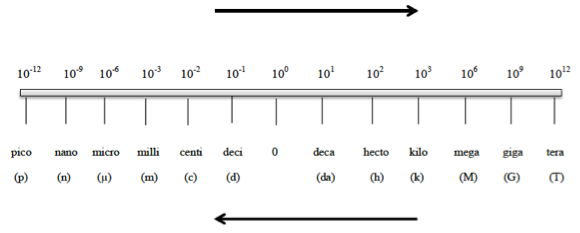# Problem: What type of quantity (for example, length, volume, density) do the following units indicate.mL

###### FREE Expert Solution

We’re being asked to determine what type of quantity (for example, length, volume, density) does mL indicate.

Recall that the SI units for length volume and density are given as:

• length → m
• Volume  → L or m3
• density → kg/cm3

Also recall that metric Prefixes are “labels” that can be placed in front of base units. Whenever using the metric prefix chart, remember that “1” is associated with the metric prefixThis means that mL is L with a metric prefix m: (m)L, therefore:

98% (152 ratings)###### Problem Details

What type of quantity (for example, length, volume, density) do the following units indicate.

mL